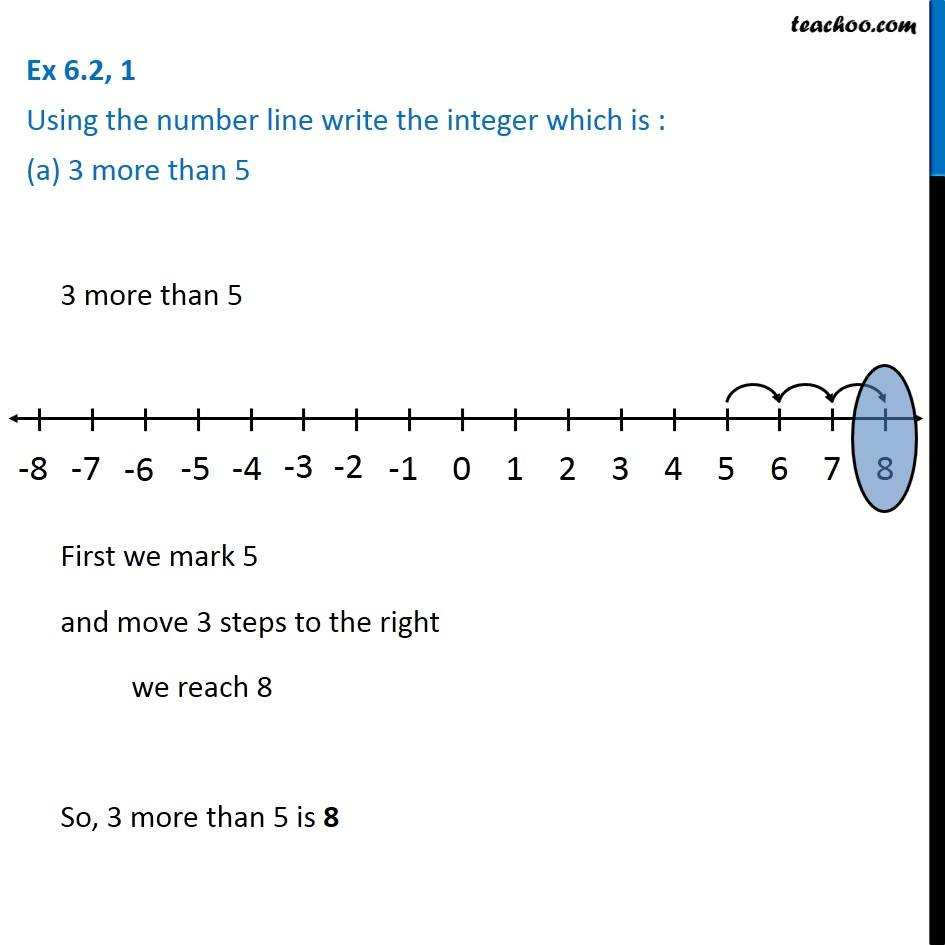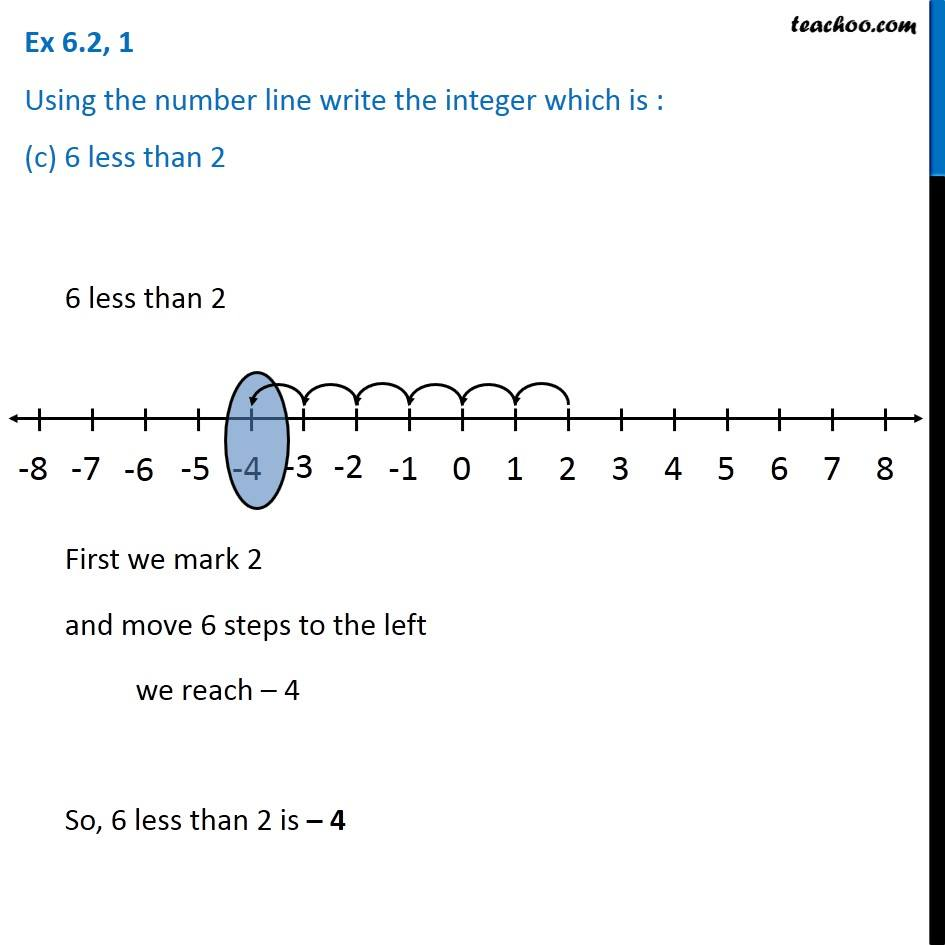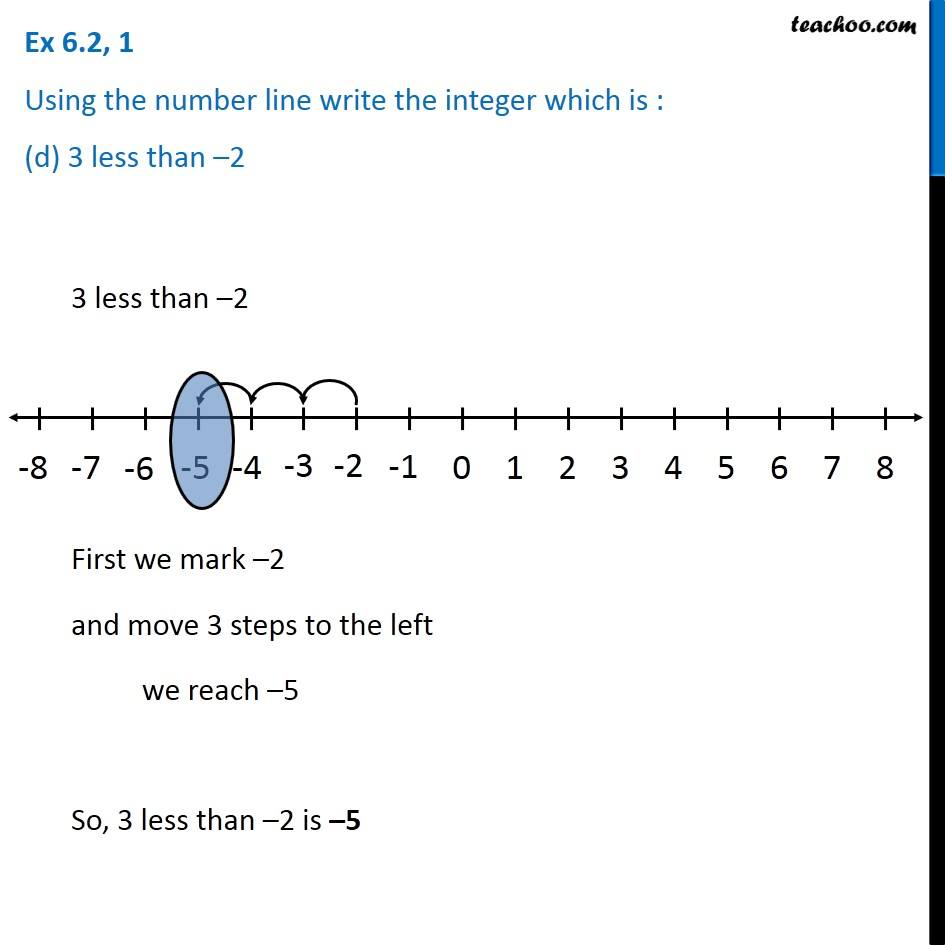1. Chapter 6 Class 6 Integers
2. Concept wise
3. Addition of integers using number line

Transcript

Ex 6.2, 1 Using the number line write the integer which is : (a) 3 more than 5 3 more than 5 First we mark 5 and move 3 steps to the right we reach 8 So, 3 more than 5 is 8 Ex 6.2, 1 Using the number line write the integer which is : (b) 5 more than –5 5 more than −5 First we mark –5 and move 5 steps to the right we reach 0 So, 5 more than –5 is 0 Ex 6.2, 1 Using the number line write the integer which is : (c) 6 less than 2 6 less than 2 First we mark 2 and move 6 steps to the left we reach – 4 So, 6 less than 2 is – 4 Ex 6.2, 1 Using the number line write the integer which is : (d) 3 less than –2 3 less than –2 First we mark –2 and move 3 steps to the left we reach –5 So, 3 less than –2 is –5

Addition of integers using number line